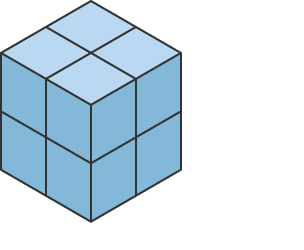## Poll

 I love math!16 votes (50%) Math is great.12 votes (37.5%) My religion is mathology.5 votes (15.62%) Women didn't speak to me until I was 30.2 votes (6.25%) Total eclipse reminder -- 04/08/202410 votes (31.25%) I steal cutlery from restaurants.3 votes (9.37%) I should just say what's on my mind.6 votes (18.75%) Who makes up these awful names for pandas?5 votes (15.62%) I like to touch my face.10 votes (31.25%) Pork chops and apple sauce.7 votes (21.87%)

32 members have voted

smoothgrhJoined: Oct 26, 2011
• Posts: 797
September 10th, 2021 at 8:53:23 PM permalink
Can someone help me because I'm terrible at math?

I just won a play money poker tournament, and my stats went from 33.5% tournaments won to 33.8% — approximately how many tournaments have I played?

I appreciate your magical mathematical minds! (It's science, but it seems like magic!)
ChesterDogJoined: Jul 26, 2010
• Posts: 964
Thanks for this post from:September 10th, 2021 at 9:23:52 PM permalink
Quote: smoothgrh

Can someone help me because I'm terrible at math?

I just won a play money poker tournament, and my stats went from 33.5% tournaments won to 33.8% — approximately how many tournaments have I played?

I appreciate your magical mathematical minds! (It's science, but it seems like magic!)

Let w = number of wins; N = number of tournaments.

Then:
w / N = 0.335
(w + 1) / (N + 1) = 0.338

Solution:
w = 73.9; N = 220.7

It looks like you had won about 74 out of 221 tournaments. And then you won another to make your record 75 out of 222.

(Check: 74 / 221 = 33.48%; 75 / 222 = 33.78%.)
ThatDonGuyJoined: Jun 22, 2011
• Posts: 4926
September 11th, 2021 at 10:12:57 AM permalink
Quote: Ace2

Thanks to ThatDonGuy for his simulation. However, I believe there is a straightforward way to calculate this formulaically. I'm still thinking of a way...the hard part is how to define the beginning/end of a "round" since new bets are being made often.

Is there a way of calculating the SD without being able to establish a finite set of profits, and a probability for each one, in any given round?

I am assuming a round begins when you have no outstanding points (i.e. either you rolled a 7, or you only had one point outstanding and you just made it).
If your first roll is a 5, then you roll any combination of 2s, 3s, 5s, 11s, and 12s, you remain in the "one point of 5" state, but the profit value can be pretty much anything. (For example, if the rolls are 5, 5, 12, then you made \$700 on the first five and lost \$100 on the 12, so your current profit is \$600; if they are 5, 11, 3, 5, 11, you made \$100 on the first 11, lost it back on the 3, made \$700 on the second 5, and made another \$100 on the 11, so your current profit is \$800. In both cases, after every roll, you had one point of 5, but no second point.)
GialmereJoined: Nov 26, 2018
• Posts: 2052
September 23rd, 2021 at 10:28:14 AM permalinkArrange n^3 small cubes into one large cube. Then paint one or more of the large cube's faces. How many small cubes are there (or "What is n"), and which large faces were painted if we find:

a) Exactly 52% of the small cubes have paint on at least one face.

b) Exactly 36% of the small cubes have paint on at least one face.

c) Exactly 8% of the small cubes have paint on at least one face.Have you tried 22 tonight? I said 22.
ThatDonGuyJoined: Jun 22, 2011
• Posts: 4926
September 23rd, 2021 at 11:47:33 AM permalink
Quote: Gialmere

Arrange n^3 small cubes into one large cube. Then paint one or more of the large cube's faces. How many small cubes are there (or "What is n"), and which large faces were painted if we find:

a) Exactly 52% of the small cubes have paint on at least one face.

b) Exactly 36% of the small cubes have paint on at least one face.

c) Exactly 8% of the small cubes have paint on at least one face.

If one face is painted, then n^2 of the cubes are painted

If two faces are painted, and
(a) they do not share an edge, then 2 n^2 of the cubes are painted;
(b) they share an edge, then 2 n^2 - n of the cubes are painted

If three faces are painted, and
(a) two of them are opposite, then the third shares separate edges with each of them, so 3 n^2 - 2 n of the cubes are painted;
(b) none of them are opposite, then they share a vertex, and 3 n^2 - 3 n + 1 of the cubes are painted

If four faces are painted, then either:
(a) they form a "ring", and 4 n^2 - 4 n cubes are painted, or
(b) one shares edges with each of the other three, so 4 n^2 - 5 n + 2 of the cubes are painted

If five faces are painted, then 6 n^2 - (n - 2)^2 = 5 n^2 + 4 n - 4 of the cubes are painted

If six faces are painted, then n^3 - (n - 2)^3 = 6 n^2 - 12 n + 8 of the cubes are painted

52% is 125 small cubes; three sides that do not share a vertex were painted